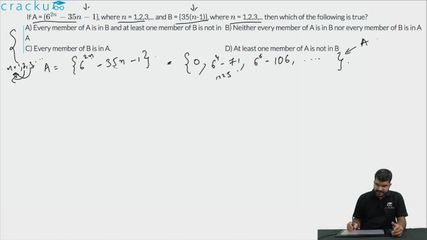Question 89

# If A = {$$6^{2n} -35n - 1$$}, where $$n$$ = 1,2,3,... and B = {35($$n$$-1)}, where $$n$$ = 1,2,3,... then which of the following is true?

Solution

If we carefully observe set A, then we find that $$6^{2n} -35n - 1$$ is divisible by 35. So, set A contains multiples of 35. However, not all the multiples of 35 are there in set A, for different values of $$n$$.
For $$n = 1$$, the value is 0, for $$n = 2$$, the value is 1225 which is the 35th multiple of 3.
If we observe set B, it consists of all the multiples of 35 including 0.
So, we can say that every member of set A will be in B while every member of set B will not necessarily be in set A.
Hence, option A is the correct answer.

### View Video Solution• All Quant CAT Formulas and shortcuts PDF
• 30+ CAT previous papers with solutions PDF